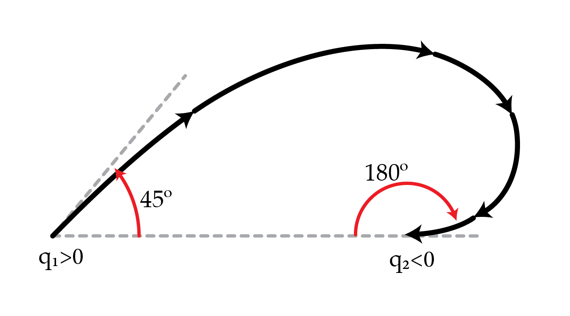# Tracing Electric Field LinesConsider two charges $q_{1}>0$ and $q_{2}<0$. An electric field line exits the positive charge $q_{1}$ at $45$ degrees and enters the negative charge $q_2$ at $180$ degrees. What is the ratio $\frac{|q_{1}|}{|q_{2}|}$?

×